+270
2.7 to 1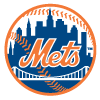Pete Alonso
18.2% implied probability

+400
4 to 1Aaron Judge
13.4% implied probability

+1500
15 to 1Mike Trout
4.2% implied probability

+1600
16 to 1Jorge Soler
4.0% implied probability

+1600
16 to 1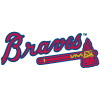Matt Olson
4.0% implied probability

+2100
21 to 1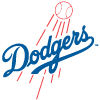Max Muncy
3.1% implied probability

+2500
25 to 1Kyle Schwarber
2.6% implied probability

+2500
25 to 1Rafael Devers
2.6% implied probability

+2500
25 to 1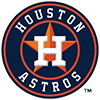Yordan Alvarez
2.6% implied probability

+2500
25 to 1Shohei Ohtani
2.6% implied probability

+3000
30 to 1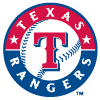Adolis Garcia
2.2% implied probability

+3000
30 to 1Fernando Tatis
2.2% implied probability

+3500
35 to 1Ronald Acuna
1.9% implied probability

+5500
55 to 1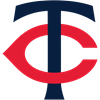Joey Gallo
1.2% implied probability

+5500
55 to 1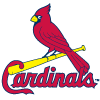Nolan Gorman
1.2% implied probability

+6000
60 to 1Austin Riley
1.1% implied probability

+6000
60 to 1Rowdy Tellez
1.1% implied probability

+6500
65 to 1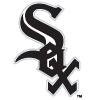Luis Robert
1.0% implied probability

+7000
70 to 1Byron Buxton
0.9% implied probability

+7000
70 to 1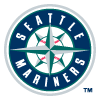Julio Rodriguez
0.9% implied probability

+7000
70 to 1Juan Soto
0.9% implied probability

+7000
70 to 1Christian Walker
0.9% implied probability

+7000
70 to 1Hunter Renfroe
0.9% implied probability

+8500
85 to 1Anthony Rizzo
0.8% implied probability

+8500
85 to 1Nolan Arenado
0.8% implied probability

+8500
85 to 1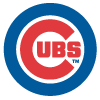Christopher Morel
0.8% implied probability

+8500
85 to 1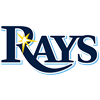Josh Lowe
0.8% implied probability

+8500
85 to 1Vladimir Guerrero
0.8% implied probability

+8500
85 to 1Randy Arozarena
0.8% implied probability

+8500
85 to 1Yandy Diaz
0.8% implied probability

+9000
90 to 1Paul Goldschmidt
0.7% implied probability

+9000
90 to 1Patrick Wisdom
0.7% implied probability

+10000
100 to 1Ozzie Albies
0.7% implied probability

+10000
100 to 1Bo Bichette
0.7% implied probability

+10000
100 to 1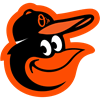Ryan Mountcastle
0.7% implied probability

+10000
100 to 1Willy Adames
0.7% implied probability

+10000
100 to 1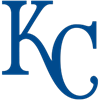Salvador Perez
0.7% implied probability

+10000
100 to 1Mookie Betts
0.7% implied probability

+15000
150 to 1Brent Rooker
0.4% implied probability

+15000
150 to 1Matt Chapman
0.4% implied probability

+15000
150 to 1C.J. Cron
0.4% implied probability

+15000
150 to 1Vinnie Pasquantino
0.4% implied probability

+15000
150 to 1Cody Bellinger
0.4% implied probability

+15000
150 to 1Anthony Santander
0.4% implied probability

+15000
150 to 1Manny Machado
0.4% implied probability

+15000
150 to 1Sean Murphy
0.4% implied probability

+15000
150 to 1Kyle Tucker
0.4% implied probability

+20000
200 to 1Jack Suwinski
0.3% implied probability

+20000
200 to 1Jake Burger
0.3% implied probability

+20000
200 to 1Joc Pederson
0.3% implied probability

+20000
200 to 1Jazz Chisholm
0.3% implied probability

+20000
200 to 1J.T. Realmuto
0.3% implied probability

+20000
200 to 1Francisco Lindor
0.3% implied probability

+20000
200 to 1Wander Franco
0.3% implied probability

No changes have been recorded yet.
+20000
200 to 1Xander Bogaerts
0.3% implied probability

+20000
200 to 1George Springer
0.3% implied probability

+20000
200 to 1Will Smith
0.3% implied probability

+20000
200 to 1Carlos Correa
0.3% implied probability

+20000
200 to 1Teoscar Hernandez
0.3% implied probability

+20000
200 to 1Corey Seager
0.3% implied probability

+20000
200 to 1Jose Ramirez
0.3% implied probability

+20000
200 to 1Marcus Semien
0.3% implied probability

+20000
200 to 1Kris Bryant
0.3% implied probability

+20000
200 to 1Alex Bregman
0.3% implied probability

+20000
200 to 1Josh Naylor
0.3% implied probability

+20000
200 to 1Freddie Freeman
0.3% implied probability

+20000
200 to 1Trea Turner
0.3% implied probability

+20000
200 to 1Brandon Lowe
0.3% implied probability

+20000
200 to 1Ryan McMahon
0.3% implied probability

+20000
200 to 1Bryan Reynolds
0.3% implied probability

+20000
200 to 1MJ Melendez
0.3% implied probability

+20000
200 to 1Eloy Jimenez
0.3% implied probability

+20000
200 to 1Daulton Varsho
0.3% implied probability

+20000
200 to 1Nathaniel Lowe
0.3% implied probability

+20000
200 to 1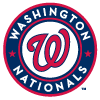Joey Meneses
0.3% implied probability

+20000
200 to 1Cal Raleigh
0.3% implied probability

+20000
200 to 1Andrew Vaughn
0.3% implied probability

+20000
200 to 1Adam Duvall
0.3% implied probability

+20000
200 to 1Bobby Witt
0.3% implied probability

+20000
200 to 1Eugenio Suarez
0.3% implied probability

+20000
200 to 1Giancarlo Stanton
0.3% implied probability